## Mathematica Assignment

I had a feeling once about mathematics – that I saw it all. Depth beyond depth was revealed to me – the Byss and the Abyss. I saw – as one might see the transit of Venus or even the Lord Mayor’s Show – a quantity passing through infinity and changing its sign from plus to minus. I saw exactly why it happened and why tergiversation was inevitable – but it was after dinner and I let it go.
Winston Churchill
Anyone who cannot cope with mathematics is not fully human. At best he is a tolerable subhuman who has learned to wear shoes, bathe and not make messes in the house.
Time Enough for Love
Robert Heinlein

### 1 Introduction

Computational tools beyond handled calculators are now a necessary part of any physicists repertoire. A wide variety of tools are available including computer codes that are written to solve specific problems, mainstream software tools (such as spreadsheets and databases) which can be applied to physics problems and finally mathematical tools (such as Mathematica, Matlab, Maple, Mathcad, Sage et cetera). We will make use of tools of all of these types in this course. The purpose of this exercise is to introduce you to one of these mathematical tools, Mathematica. Mathematica distinguishes itself from many of its competitors with its abilities to do exact symbolic, as well as numerical, calculations. These abilities allow Mathematica to, for example, give the solution ofwhile most other programs would only be able to solve problems such as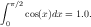Keep these differences in mind while doing the exercises below.

In this exercise, you will first work through a Mathematica tutorial to get an idea of Mathematica’s abilities. Then you will use Mathematica to work a more complicated physics problem.

### 2 Tutorial

Start up Mathematica (this tutorial can be run on any OS that Mathematica works on).

For this tutorial you will have two choice. You can you the old-fashioned text-based tutorial or a video screencast. It is up to you which one to try — you should get similar information from each.

If you want to use the text based tutorial, find and open up the “Ten Minute Tutorial” – there should be a link to it off of this lab (or the lab web site). Do not let the name fool you — it should take you much more than 10 minutes. Note that when you download Mathematica files you will often have to right click on them and “Save as ...”, then open them up with Mathematica.

If you want to use the screencast, go to the “Hands on Start to Mathematica”

http://www.wolfram.com/broadcast/screencasts/handsonstart/. If you do the screencast when other people are around, please make sure that you use headphones. I will not be responsible for any violence inflicted on you by other people if you blast this screencast in a public lab.

As you are working through the tutorial feel free to change things in the examples. Try out different numbers, functions, et cetera so that you have a better idea of how Mathematica works. Save a copy of the the tutorial in your home directory so that you can keep track of the changes you make while working through the tutorial.

Complete the following exercises based on the tutorial. For this exercise, you can either do things on paper and hand-in a paper copy, or upload your results to the course Canvas site. Where appropriate print out (or save to a separate Mathematica sheet) plots and segments of Mathematica code showing your answers. If you decide to upload the assignment, please upload the notebook (.nb) file and also a pdf copy of file. (Print the file to a pdf.)

The easiest way to answer the following questions is probably to have two Mathematica windows open. Keep the tutorial open in one window, and do your calculations in separate window. In your calculations window you can copy and paste all of the questions below. Then you complete each calculation under the corresponding question. When you are done you can just print the results from your window (or upload them to Canvas). Also, do not forget to include a written answer where one is requested.

The page numbers below refer to where you will find similar calculations in the text tutorial.

1. (p. 3) Calculate 2131 and 21.31 power. What is the difference between these results? Why are they different? Which answer would most other mathematical tools give? Why?
2. (p. 5) Get a numerical expression for the first 40 digits of eπ.
3. (p. 6) Expand and simplify (a + b)(a - c)(b - d) + (d - c)(b - a)(c + a).
4. (p. 8) Plot sinx, sinhx, and sin(sinh(x)) from 0 to 5 on the same plot. Also plot in three dimensions the function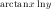over the range from 0 to 5 for x and over the range from 1 to 3 for y.
5. (p. 9) Calculate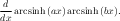The computeIntegrate the result. Finally, numerically integrate6. (p. 11) Solve the system of equations x2 - y2 = a and x + 3y = b.
7. (p. 13) Solve and plot the solution to the differential equation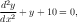where y(0) = 0 and y(0) = 0.

### 3 Nonlinear Pendulum

In this part you will put to use some of the Mathematica commands that you learned above. In deriving the motion of a simple pendulum (as seen on the right), using torques (or forces) leads to the equation of motion: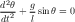(1)In order to get this equation in the form of the simple harmonic oscillator equation, we typically assume that the angle θ is small so that sinθ θ, which results in: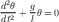(2)

In this exercise you will explore how justified this approximation is. In some parts of this exercise you may have to force Mathematica to make more precise calculations. You may have to use the Accuracy, WorkingPrecision, AccuracyGoal, and PrecisionGoal statements to get useful results. For the purposes of this exercise we will set g/l = 1 to simplify the math. We will also assume that the pendulum always starts out at rest (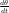(t = 0) = 0).

1. Find how varying the starting angle alters the angular frequency of the pendulum when you don’t make the small angle approximation. Use Mathematica to solve the pendulum differential equation above for the case where the initial pendulum amplitude (angle) is 1 radian. From this solution, find the pendulum frequency (1/period) for this case. You will probably want to use the NDSolve and FindRoot functions to do this.

Next, solve this equation for initial amplitudes from 0 to π∕2 radians and then plot the pendulum frequency versus pendulum (angle) amplitude for your results. Also, on the same figure include a plot of what the angular frequency versus amplitude is assuming the pendulum is a simple harmonic oscillator. Take enough points so that you get a fairly smooth curve. You don’t have to use Mathematica to plot your results — any plotting program is fine.

2. Calculate at what amplitude the angular frequency differs from the ideal angular frequency by 10 % ? By 1 % ? By 0.01 % ? By 0.0001 % ? What does this tell you about the accuracy of the small angle approximation? Under what conditions is this approximation valid?
3. One of the applications of pendulums is in clocks. Calculate how long it would take for a clock using this pendulum to be displaying a time that is off by a minute if the amplitude of the pendulum is 0.1? 1? 10? 30? 90?